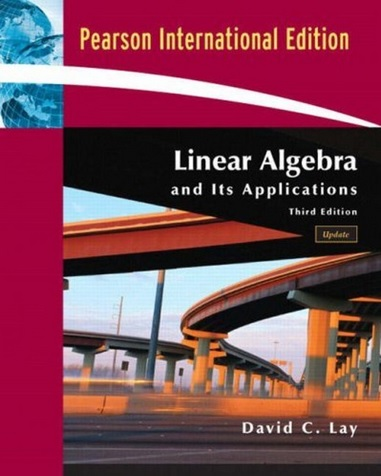Back to main page

## Linear Algebra and Its Applications

By David C. Lay. (Third Edition)These are my proposed solutions. If you find any mistakes, please let me know.

#### Chapter 1 - Linear Equations in Linear Algebra

1.1 - Systems of Linear Equations
1.2 - Row Reduction and Echelon Forms
1.3 - Vector Equations
1.4 - The Matrix Equations Ax = b
1.5 - Solution Sets of Linear Systems
1.6 - Applications of Linear Systems [Skipped]
1.7 - Linear Independence
1.8 - Introduction to Linear Transformations
1.9 - The Matrix of a Linear Transformation
1.10 - Linear Models in Business, Science and Engineering [Skipped]

#### Chapter 2 - Matrix Algebra

2.1 - Matrix Operations
2.2 - The Inverse of a Matrix
2.3 - Characterization of Invertible Matrices
2.4 - Partitioned Matrices
2.5 - Matrix Factorizations
2.6 - The Leontief Input-Output Model
2.7 - Application to Computer Graphics
2.8 - Subspaces of Rn
2.9 - Dimension and Rank

#### Chapter 3 - Determinants

3.1 - Introduction to Determinants
3.2 - Properties of Determinants
3.3 - Cramer's Rule, Volume, and Linear Transformations

#### Chapter 4 - Vector Spaces

4.1 - Vector Spaces and Subspaces
4.2 - Null Spaces, Column Spaces, and Linear Transformations
4.3 - Linearly Independent Sets; Bases
4.4 - Coordinate Systems
4.5 - The Dimensions of a Vector Space
4.6 - Rank
4.7 - Changes of Basis
4.8 - Applications to Difference Equations
4.9 - Application to Markov Chains

#### Chapter 5 - Eigenvalues and Eigenvectors

5.1 - Eigenvectors and Eigenvalues
5.2 - The Characteristic Equation
5.3 - Diagonalization
5.4 - Eigenvectors and Linear Transformations
5.5 - Complex Eigenvalues
5.6 - Discrete Dynamic Systems
5.7 - Applications to Differential Equations
5.8 - Iterative Estimates for Eigenvalues

#### Chapter 6 - Orthogonality and Least Squares

6.1 - Inner Product, Length, and Orthogonality
6.2 - Orthogonal Sets
6.3 - Orthogonal Projections
6.4 - The Gram-Schmidt Process
6.5 - Least-Squares Problems
6.6 - Application to Linear Models
6.7 - Inner Product Spaces
6.8 - Applications to Inner Product Spaces

#### Chapter 7 - Symmetric Matrices and Quadratic Forms

7.1 - Diagonalization of Symmetric Matrices